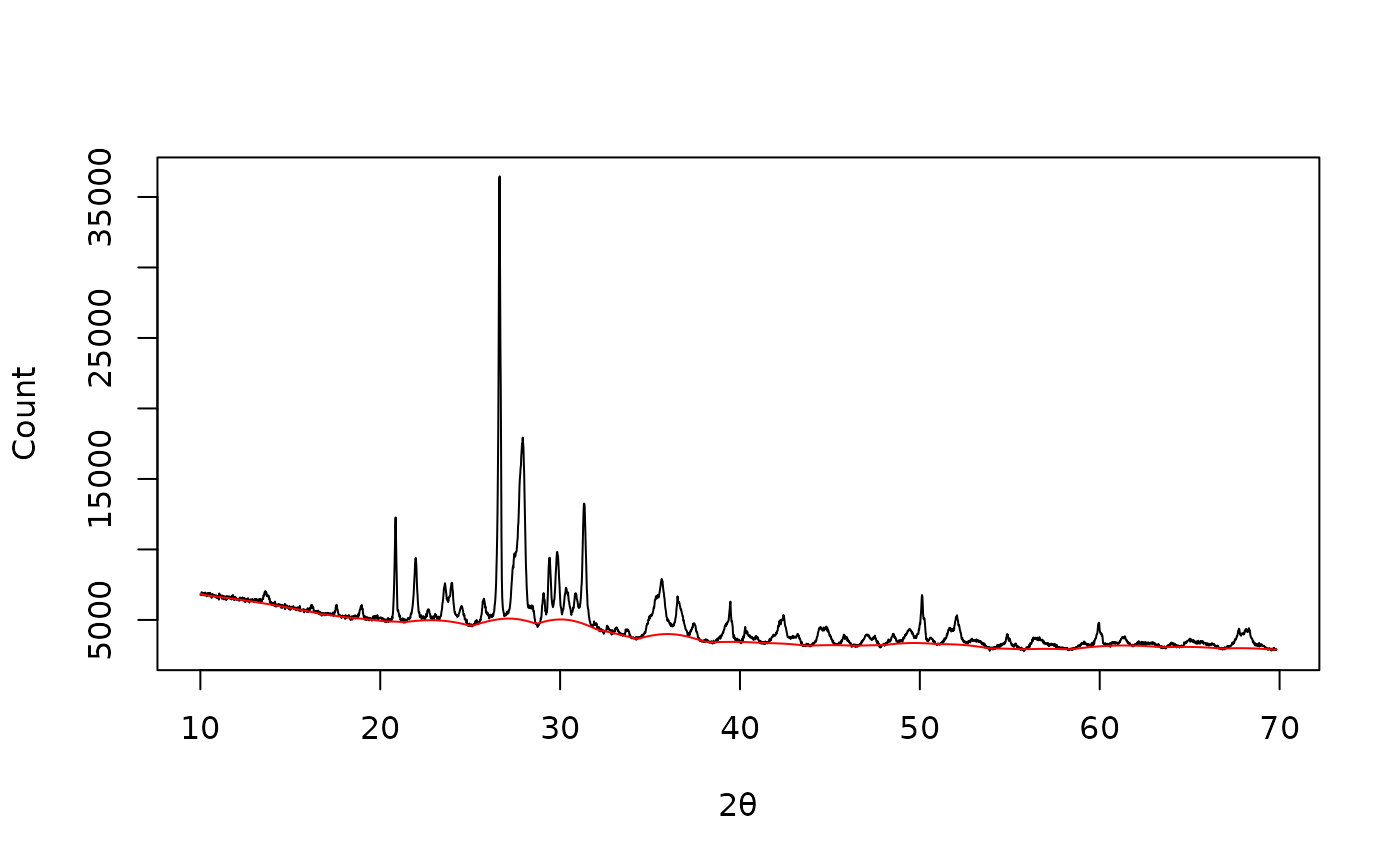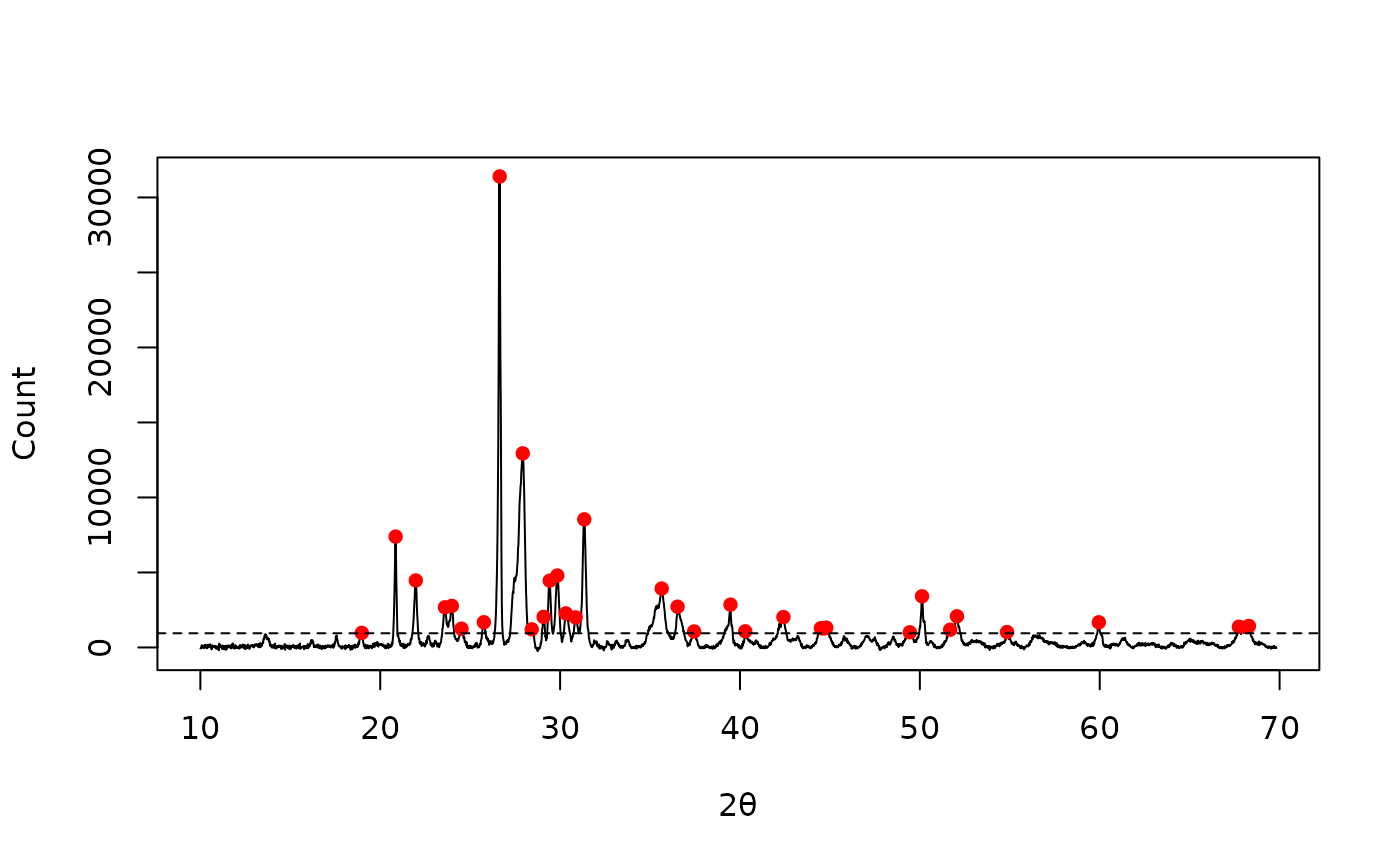Estimates the Half-Width at Half-Maximum (FWHM) for a given peak.

## Usage

peaks_fwhm(x, y, ...)

# S4 method for numeric,numeric
peaks_fwhm(x, y, center)

# S4 method for ANY,missing
peaks_fwhm(x, center)

## Arguments

x, y

A numeric vector. If y is missing, an attempt is made to interpret x in a suitable way (see grDevices::xy.coords()).

...

Currently not used.

center

A numeric value giving the peak position in x units.

## Value

A numeric value.

## Details

It tries to get the smallest possible estimate.

Other peaks detection methods: peaks_find()

N. Frerebeau

## Examples

## X-ray diffraction
data("XRD")

## 4S Peak Filling baseline
baseline <- baseline_peakfilling(XRD, n = 10, m = 5, by = 10, sparse = TRUE)

plot(XRD, type = "l", xlab = expression(2*theta), ylab = "Count")
lines(baseline, type = "l", col = "red")## Correct baseline
XRD <- signal_drift(XRD, lag = baseline, subtract = TRUE)

## Find peaks
peaks <- peaks_find(XRD, SNR = 3, m = 11)

plot(XRD, type = "l", xlab = expression(2*theta), ylab = "Count")
lines(peaks, type = "p", pch = 16, col = "red")
abline(h = attr(peaks, "noise"), lty = 2) # noise threshold## Half-Width at Half-Maximum
x <- seq(-4, 4, length = 1000)
y <- dnorm(x)

peaks_fwhm(x, y, center = 0) # Expected: 2 * sqrt(2 * log(2))
#>  2.354354